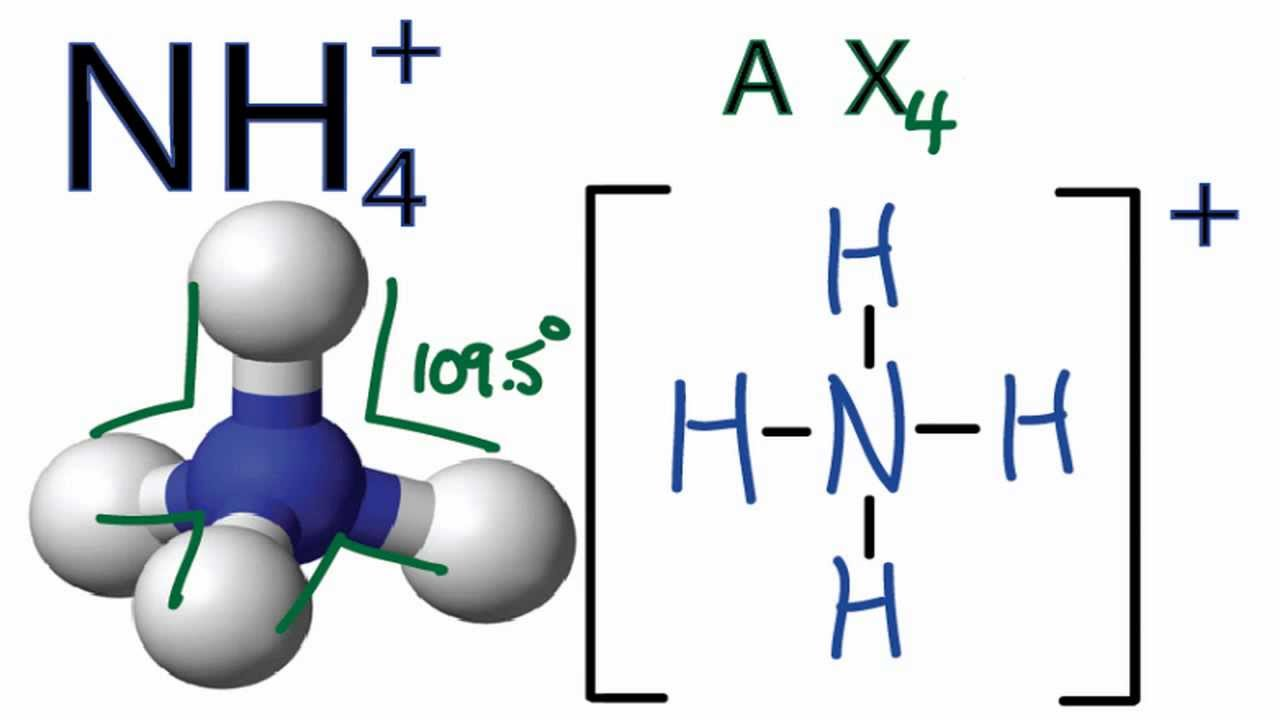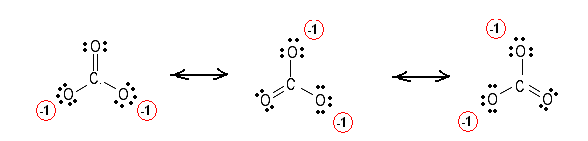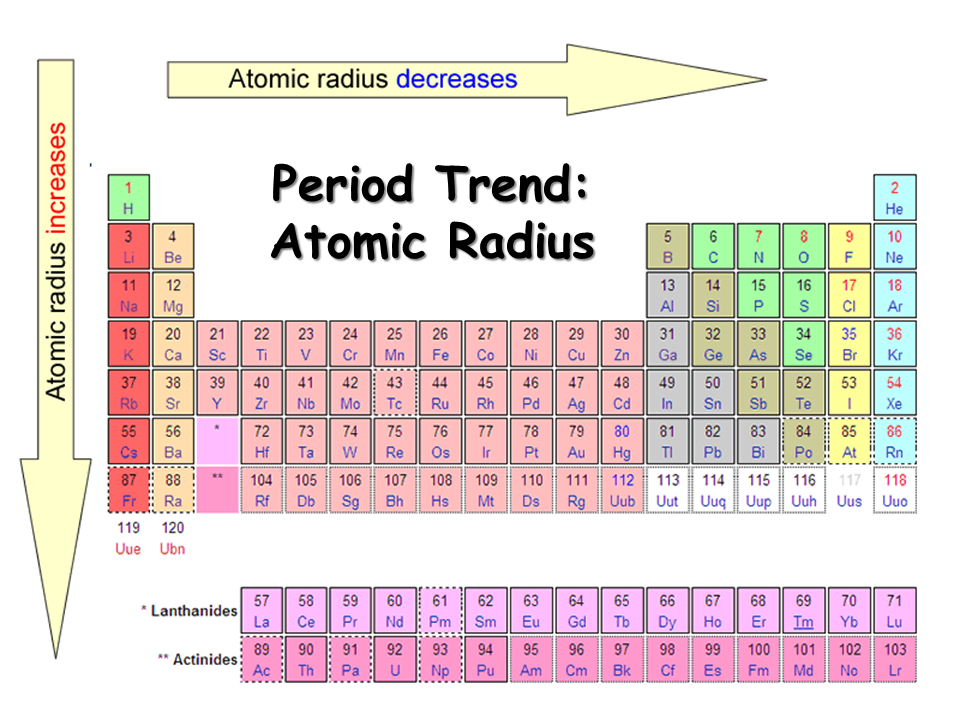Resources:

Have you found videos, websites, or explanations that helped you understand this chapter? Let us know and we'll add them to "Resources" part of this page for other students to use.

Question 1

How many valence electrons does boron have?

Explanation

[He] 2s2 2p1

The valence electrons are the ones in the highest nth energy level. In this case it is the second energy level. This means that there are 3 electrons

Question 2

How many valence electrons does magnesium have?

Explanation

Ne 3s2

The valence electrons are the ones in the highest nth energy level.

Question 3

How many valence electrons does bromine have?

Explanation

[Ar] 3d10 4s2 4p5

The valence electrons are the ones in the highest nth energy level.

Question 4

How many valence electrons does argon have?

Explanation

[Ne] 3s2 3p6

The valence electrons are the ones in the highest nth energy level.

Question 5

Which of the following statements about Lewis Structures is FALSE?

Select one:Explanation

Carbon only makes 4 bonds

Question 6

Write the Lewis structure for H2O and check those statements which are correct.

Select one or more:ExplanationO is the central atom

H2O : 2 + 6 = 8

oxygen has 2 lone pairs

Question 7

Write the Lewis structure for NH4 +1  and check those statements which are correct.

Select one or more:Explanation

NH4 +1 =  5 + 4 -1 = 8 valence electronsQuestion 8

Write the Lewis structure for SiBr4 and check those statements which are correct.

Select one or more:ExplanationQuestion 9

Which structure correctly represents hydroxylamine, NH3O. Also, check those statements which are correct for the completed Lewis structure.Select one or more:Explanation

The second structure is correct because the N has a charge of zero. While the first structure has a charge of  +1 for N.Question 10

Which Lewis structure, satisfying the octet rule, correctly represents phosphorous acid, H3PO3. Also, check those statements which are correct for the completed Lewis structure satisfying the octet rule.Select one or more:Explanation

Structure II is correct because neutral charge of 0 matches the formula's charge.

H3PO3 = 3 +  5 +( 6*3 ) = 26 valence electrons

P : has one lone pairs

Question 11

Formaldehyde, H2CO, is a gas with a characteristic pungent odor. Draw its Lewis structure. Which statements are correct regarding its Lewis structure?

Select one or more:ExplanationQuestion 12

Draw the Lewis structure for hydogen cyanide, HCN. Which statements are correct regarding its Lewis structure?

Select one or more:ExplanationQuestion 13

Draw the Lewis structures for the carbonate ion, CO32-. Which statements are correct regarding its Lewis structures?

Select one or more:ExplanationQuestion 14

Draw the Lewis structure for the iodine dioxide difluoride ion, IO2F2-. Which statements are correct regarding its Lewis structure? (Note, all the atoms are bonded to the central I atom.)

Steric Number

Select one or more:ExplanationQuestion 15

Draw the Lewis structure and shape for the ammonia, NH3. Which of the following statements are correct.

Select one or more:ExplanationN : 3 bonds + 1 lone pair =  trigonal pyramidal

Question 16

Draw the Lewis structure and shape for PCl5. Which of the following statements are correct.

Select one or more:ExplanationP : 5 bonds  +  0 lone pair = trigonal biprymidal

Question 17

Which statements are correct for carbon dioxide?

Select one or more:ExplanationC : 2 bonds = linearQuestion 18

Complete the Lewis structure for the following hydrocarbon. Which statements are correct for this compound?Select one or more:Explanation

C1: 4 bonds + 0 lone pair = 4

C2: 2 bonds = 2

C4 : 3bonds = 3

O1: 1 bond + 2 lone pair = 3

O2: 2 bonds + 2 lone pair = 4Question 19

Which of the following ionic compounds has the highest melting point?

Select one:Explanation

Smallest radius = strongest bonds = high melting point

Highest Product of Charge = high melting pointQuestion 20

Which of the following molecules are nonpolar?

(You need to draw the Lewis structure for each molecule unless you already know by analogy. For example, NH3 and PH3 have the same shape because P is below N on the periodic table, so they have the same number of valence electrons.)

Select one or more:Explanation

= tetrahedral = nonpolar (symmetrical )

SF6 = octahedral = nonpolar (symmetrical)Question 21

Which of the following molecules have dipole-dipole forces between different molecules? (Lewis structures may need to be drawn.)

Select one or more:Explanation

Rule of Thumb: All polar molecules have dipole -dipole attraction

HCl = polar (linear) uneven electronegativity

BI2Cl = polar

: polar

Question 22

Which of the following molecules have H-bonding and thus a higher than expected melting and boiling point?

Select one or more:Explanation

H bonds are with F, O, N

H bonds are one of the strongest types of bond

Question 23

For each of the following molecules pick the one with the greater London forces and hence higher melting and boiling point?

Select one or more:Explanation

London forces (Dispersion Forces) are the strongest for nonpolar molecules that do not have hydrogen bond

Question 24

Check the correct statements about carbon dioxide.Select one or more:Question 25

Calculate the new freezing point for a 0.73 m solution of CCl4 in benzene.Explanation

ΔT = m x Kf  = 0.73 x 4.90 = 3.577

T = 5.51 - 3.577 = 1.933 C

Question 26

Calculate the increase in the boiling point, ΔT, for a 0.53 m solution of sucrose (dissolves as a molecule) in water.Explanation

ΔT = m x Kb = 0.53 x .512 = 0.27

Question 27

Which 0.06 m aqueous solution should have the greatest increase in boiling point?
Select one:Explanation

Large amount of heat which is needed to break the strong ionic bond.

Question 28

A strong acid is spilled on your hands. Which procedure works best?

Select one:Explanation

Base can neutralize an Acid

Question 29

What is the correct procedure for mixing an acid and water and why?

Select one:Explanation

Acid is more dense than water.